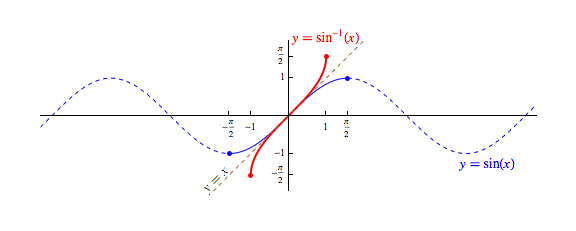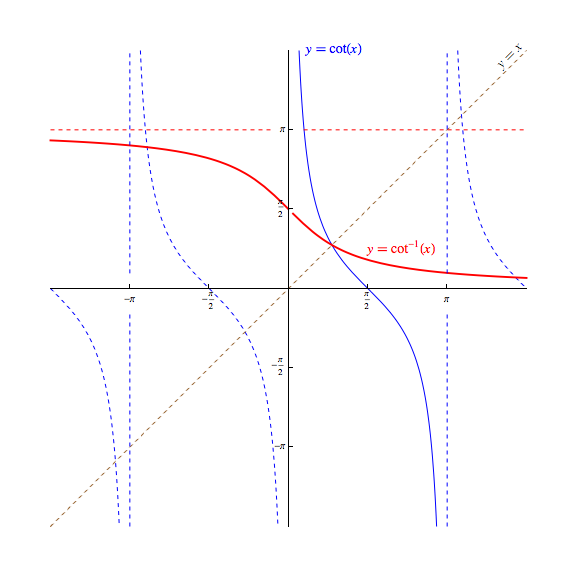## Inverse Trigonometric Functions

We will often have need to ask the question: "For what angle does the sine (or cosine, tangent, ...) equal such-and-such?"

Such a question is normally answered by finding and applying an inverse function. However, due to their periodic nature, none of the six trigonometric functions pass the horizontal line test, and as such, none of these functions have a true inverse function.

That said, we can find something almost as useful...

Let us start by considering $\sin x$. If we restrict the domain of the sine function to $[-\pi/2,\pi/2],$ the resulting function will pass the horizontal line test, and consequently has an inverse. The graph of this inverse is shown below in red.Restricting the domain to $[-\pi/2,\pi/2]$ is useful on a couple of fronts. Not only do we produce an invertible function, but we also produce one that has the exact same range as the sine function with an unrestricted domain.

Additionally, while there are several intervals that do both of these things (e.g., $[\pi/2,3\pi/2]$, $[-3\pi/2,-5\pi/2]$, etc.), the interval $[-\pi/2,\pi/2]$ minimizes the magnitudes of the angles involved.

The function drawn above in red is called the arcsine function and is denoted either by $\arcsin x$, or $\sin^{-1} x$. This function behaves in many ways (but not all) as an inverse to the unrestricted domain version of $\sin x$.

For instance,

• Like most inverse function pairs, the domain of $\sin^{-1} x$ is identical to the range of the $\sin x$, both being $[-1,1]$.   However, the range of $\sin^{-1} x$ is only $[\pi/2,\pi/2]$, a small subset of the domain of the $\sin x$.

• For any $x$ in the domain of $\sin^{-1}$, we have $\sin(\sin^{-1} x) = x$. However, $\sin^{-1} (\sin x) = x$ is true only if $x \in [-\pi/2,\pi/2]$ and false otherwise!

In a like manner, the remaining five trigonometric functions have "inverses":

The arccosine function, denoted by $\arccos x$ or $\cos^{-1} x$ is the inverse to the cosine function with a restricted domain of $[0,\pi]$, as shown below in red.The arctangent function, denoted by $\arctan x$ or $\tan^{-1} x$ is the inverse to the tangent function with a restricted domain of $(-\pi/2,\pi/2)$, as shown below in red.Similarly, we define the arccotangent function, denoted by $\textrm{arccot } x$ or $\cot^{-1} x$ to be the inverse to the cotangent function with a restricted domain of $(0,\pi)$, as shown below in red.

Interestingly, our choice for which "piece" of the cotangent function to invert to produce the arccotangent function is not universally agreed upon. The advantage of our selection of $(0,\pi)$ is that the resulting arccotangent function is continuous and defined everywhere, and behaves in a manner more consistent with that seen in the related $\tan^{-1}$ function.We also define the arcsecant function, denoted by $\textrm{arcsec } x$ or $\sec^{-1} x$ to be the inverse to the secant function with a restricted domain of $[0,\pi]$, as shown below in red.

Here again, there is not universal agreement that $[0,\pi]$ should be the "piece" of the secant function that should be inverted to produce the arcsecant function. James Stewart's Single Variable Calculus, for instance, uses $[0,\pi/2) \cup [\pi,3\pi/2)$ instead. Both choices result in a discontinuous graph, however ours minimizes the magnitudes of the angles involved.Lastly, we define the arccosecant function, denoted by $\textrm{arccsc } x$ or $\csc^{-1} x$ to be the inverse of the cosecant function with a restricted domain of $[-\pi/2,0) \cup (0,\pi/2]$, as shown below in red.

Once again, some authors define the arccosecant to be the inverse of a different "piece" of the cosecant function, such as $(0,\pi/2] \cup (\pi,3\pi/2]$. As was the case with the arcsec, both choices result in a discontinuous graph. Our choice, however, again minimizes the magnitudes of the angles involved.